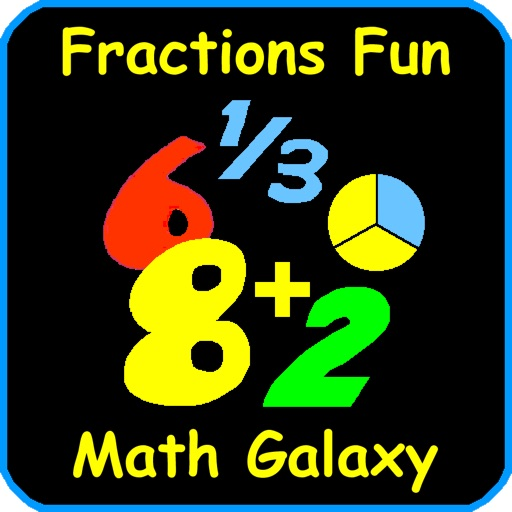## Math Galaxy Fractions Fun is a comprehensive fractions tutorial with explanations, practice, and games that cover all fraction operations and concepts, including equivalent fractions, comparing fractions, reducing fractions, improper fractions, prime factors, greatest common factor, least common multiple, adding, subtracting, multiplying, dividing, word problems, plus geometry, English and metric measurement, graphs and probability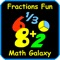# Math Galaxy Fractions Fun

by Math Galaxy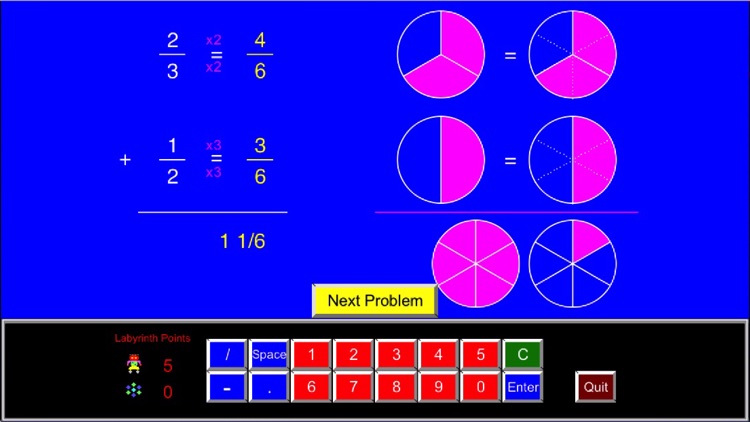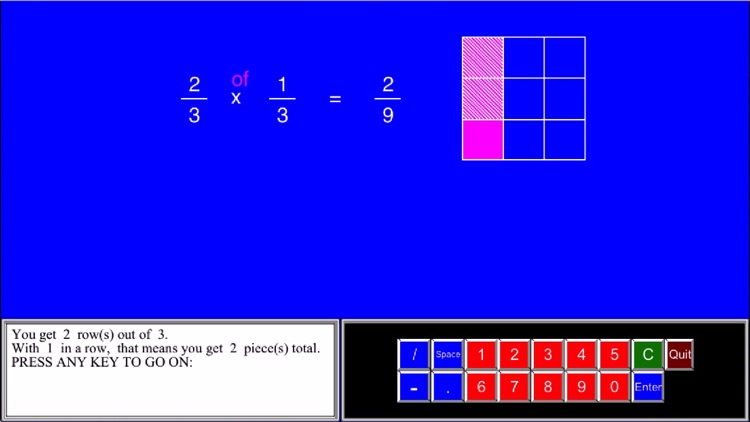Math Galaxy Fractions Fun is a comprehensive fractions tutorial with explanations, practice, and games that cover all fraction operations and concepts, including equivalent fractions, comparing fractions, reducing fractions, improper fractions, prime factors, greatest common factor, least common multiple, adding, subtracting, multiplying, dividing, word problems, plus geometry, English and metric measurement, graphs and probability.Version
1.6
Rating
NA
Size
154Mb
Genre
Education Casual
Last updated
August 21, 2017
Release date
July 25, 2012

### App Screenshots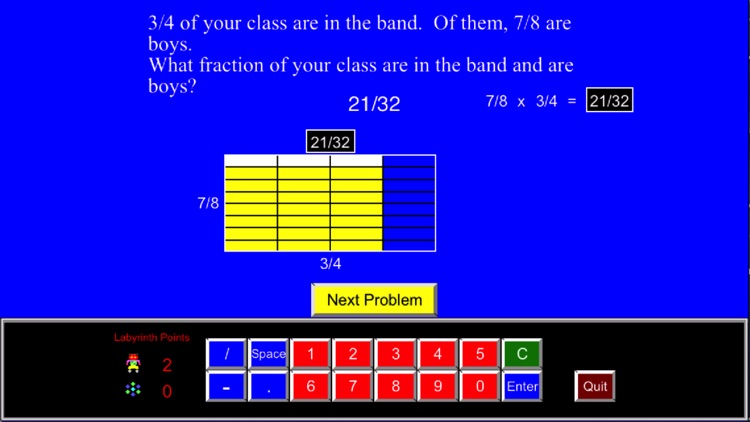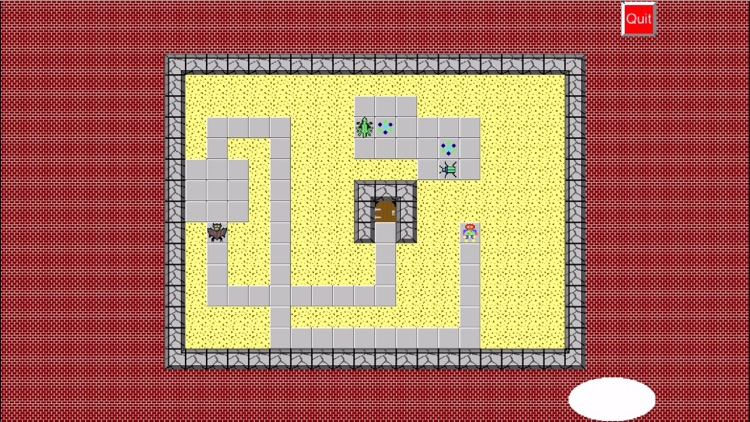### App Store Description

Math Galaxy Fractions Fun is a comprehensive fractions tutorial with explanations, practice, and games that cover all fraction operations and concepts, including equivalent fractions, comparing fractions, reducing fractions, improper fractions, prime factors, greatest common factor, least common multiple, adding, subtracting, multiplying, dividing, word problems, plus geometry, English and metric measurement, graphs and probability.

By solving problems students earn robots to use in a maze game called the Labyrinth.
Other number games include Bridge the Swamp and The Money Trail.

Math Galaxy's approach to learning math is based on the philosophy that:

(1) New ideas must be connected to a child's current network of knowledge and experience.

(2) Skills develop along a concrete-to-abstract continuum, with advanced skills developed by generalizing from more concrete experiences.

(3) A child must see a wide range of instances of a concept in order to separate out extraneous material from what is essential.

(4) A child needs to be actively engaged with new ideas and not passively entertained.

(5) Ultimately, students will be using calculators, so the goal of elementary math should not be to add or multiply long columns of numbers but to understand the basic concepts behind the operations.

Unlike most math textbooks, which throw a mass of material at the student, Math Galaxy focuses student attention on concepts sequentially and interactively, based upon the ideas behind math manipulatives (such as counting blocks) and connecting new concepts to familiar experiences. Unlike most math software, it goes beyond simple games, low-level operations or rule memorization to link familiar and concrete experiences to higher-level processes. It goes beyond physical math manipulatives by linking each physical operation to its mathematical counterpart at each step, and by allowing manipulations that are difficult to do with physical manipulatives. It stresses concepts that run throughout all of basic math to provide basic understanding rather than memorized rules.

Our goal is to provide students a foundation for analytical thinking in the modern world and for higher-level math and science.

The key to learning math is consistency. It is best taken in small doses but done consistently. Even ten minutes or ten problems a day will improve your child's understanding of math if done consistently.

"As I explored each area of the program, I became more and more impressed with the mathematical challenges it presented."

"Each of the various areas that your child can can work in has several nice functions."
"I do highly recommend Math Galaxy Fractions Fun for those who would like additional resources to supplement their math curriculum, as a tool to present a difficult curriculum in a different way, and as drill practice."

Disclaimer:
AppAdvice does not own this application and only provides images and links contained in the iTunes Search API, to help our users find the best apps to download. If you are the developer of this app and would like your information removed, please send a request to [email protected] and your information will be removed.